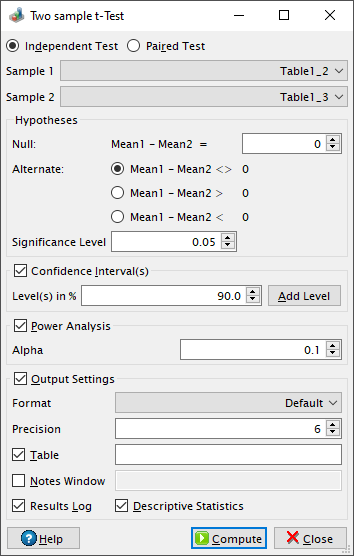# Two sample t-Test Dialog

This dialog is activated by selecting the Two Sample t-Test... command from the Statistics -> Hypothesis Testing -> menu.

A t-test is any statistical hypothesis test in which the test statistic follows a Student's t-distribution under the null hypothesis. The two sample t-test can be used to determine if two sets of data are significantly different from each other. It is assumed that the data is normally distributed. Please visit the Wikipedia article on the Student's t-test for more details about this statistical test.

Figure 5-106. Two sample t-Test dialog.The two-sample form of the t-Test compares the difference between the means (m1-m2) of the two data sets to a mtest difference specified in the null hypothesis. Setting the test difference to 0 is equivalent to testing the equality of the means. By default QtiPlot performs a two-tailed test, the default alternate hypothesis being that the difference between means and mtest are different (m1-m2 <> mtest). It is also possible to perform an upper-tailed test by choosing the alternate hypothesis that m1-m2 > mtest or a lower-tailed test (m1-m2 < mtest).

This test uses the statistic t = (m1-m2-mtest)/[Sp(1/N1+1/N2)1/2], where Sp is the pooled variance of the two samples and N1 and N2 are the sizes of the two data sets.

For an independent test Sp = [((N1-1)V1+(N2-1)V2)/(N1+N2-2)]1/2, where V1 and V2 are the sample variances. For a paired test N1 = N2 and Sp is calculated as the variance of the differences between the corresponding values from the two samples.

The test statistic is used to compute a probability (P value). For a lower-tailed test the probability is calculated using the formula p = tcdf(t, DoF), where the tcdf function calculates the lower tail of the cumulative distribution function for the Student's t-distribution with DoF degrees of freedom. For a paired test DoF = N-1, where N = N1 = N2 is the common size of the two data sets, whereas for an independent test DoF = N1+N2-2. For an upper-tailed test, the probability is calculated using the formula p = 1-tcdf(t, DoF). For a two-tailed test the probability is calculated using the formulas p = 2tcdf(t, DoF) if tcdf(t, DoF) < 0.5 and p = 2[1-tcdf(t, DoF)] otherwise.

The null hypothesis is rejected if the calculated probability is lower than the value of the Significance Level.

If the Confidence Interval(s) box is checked QtiPlot also computes a confidence interval (Lower Limit and Upper Limit) for each user defined level.